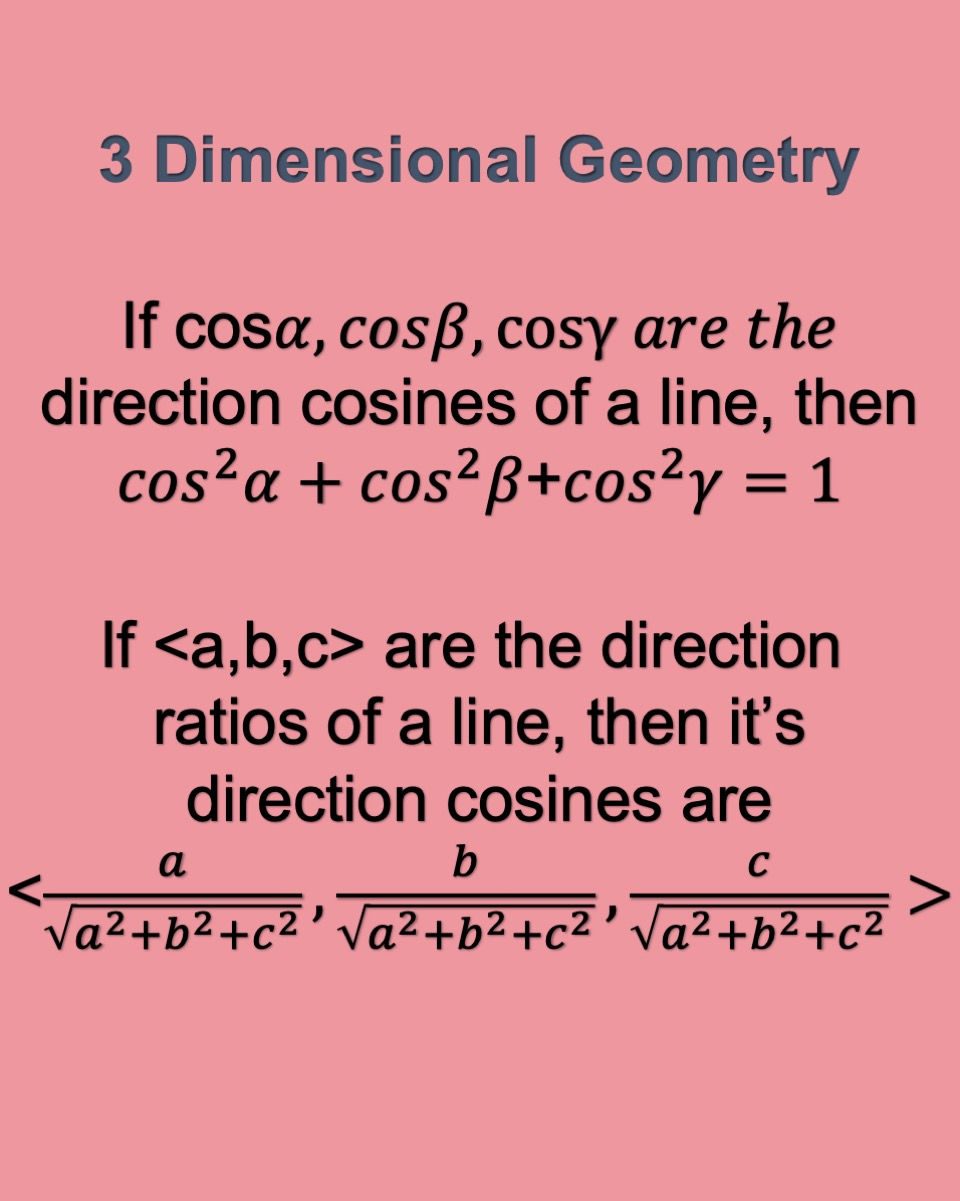top of page
Search

# Relation between direction ratios and direction cosines of a line

Presenting 2 formulas. The first cone gives you an identity relating to the square of the sum of the direction cosines of a line. The second one shows you how to calculate the direction cosines of a line, given the direction ratios. Do you know why we use this formula? Email me your answers at mathmadeeasy22@gmail.com